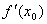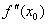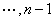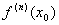1．单变量函数的极值

[极值（极大值或极小值）]若函数f (x)在点x0的双侧邻域中有定义，并且对于某邻域0<|x-x0|<δ内的一切点x，下面不等式成立：

f (x)< f (x0)    (f (x)> f (x0))

[极值存在的必要条件]假定函数f (x)在区间(a,b)内存在有限导数.若在点x0((a,b))处函数有极值，则必有

`=0                                                       (1)`

[极值存在的充分条件]

 x x < x0 x 0 x> x0 f (x)+ ― + ― 0 ― + + ― 极大值 极小值 上升 下降=00=0         k=1,0

n为偶数，则函数f (x)在点x0处有极值（当<0时有极大值，当>0时有极小值）;n为奇数，则在点x0处无极值.=0